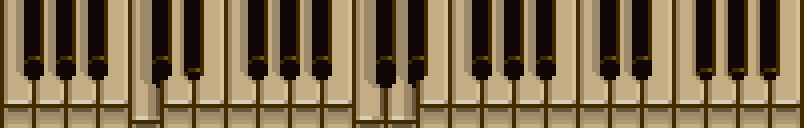# HELP

## open up the console (ctrl+shift+j on chromium) and generate your graphs and knots !

 G // this is the graph G.clearAll() // this will remove everything we have now. let n1 = G.addNode([0,2]) // add a node at [0,2] on screen let n2 = G.addNode([0,1]) // add a node at [0,1] on screen G.addEdge(n1,n2) // add an edges between the two previous nodes // there also is an useful function that adds vectors // as we can't overload operator+ in js let v = vec2add([0,1],[-1,-1]) // here is an example of it's usage let generate_mesh1 = (k_max,position) => { n = G.addNode(vec2add(position,[0,0])); for( let k=1 ; k < k_max ; k++ ) { let θ = 2*π*k/k_max + π/2 n[k] = G.addNode(vec2add(position,[cos(θ),sin(θ)])); } for( let k=1 ; k < k_max ; k++ ) { G.addEdge(n,n[k]); } for( let k=1 ; k < k_max-1 ; k++ ) { G.addEdge(n[k],n[k+1]); } } generate_mesh1(7,[0,-1])